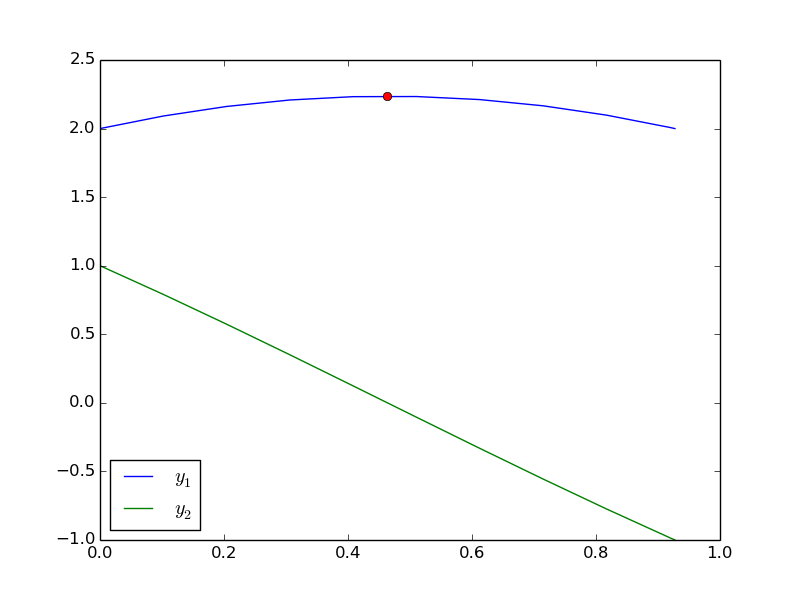## Using events in odelay with multiple equations

| categories: odes | tags: events

odelay was recently updated to support multiple odes with events. Here is an example. We want the solutions to:

\begin{align} \frac{dy_1}{dx} = y_2 \\ \frac{dy_2}{dx} = -y_1 \end{align}

with initial conditions $$y_1(0) = 2$$ and $$y_2(0) = 1$$. We want to stop the integration when $$y_2 = -1$$ and find out when $$dy_1/dx=0$$ and at a maximum.

from pycse import odelay
import matplotlib.pyplot as plt
import numpy as np

def ode(Y,x):
y1, y2 = Y
dy1dx = y2
dy2dx = -y1
return [dy1dx, dy2dx]

def event1(Y, x):
y1, y2 = Y
value = y2 - (-1.0)
isterminal = True
direction  = 0
return value, isterminal, direction

def event2(Y, x):
dy1dx, dy2dx = ode(Y,x)
value = dy1dx - 0.0
isterminal = False
direction = -1  # derivative is decreasing towards a maximum
return value, isterminal, direction

Y0 = [2.0, 1.0]
xspan = np.linspace(0, 5)
X, Y, XE, YE, IE = odelay(ode, Y0, xspan, events=[event1, event2])

plt.plot(X, Y)
for ie,xe,ye in zip(IE, XE, YE):
if ie == 1: #this is the second event
y1,y2 = ye
plt.plot(xe, y1, 'ro')

plt.legend(['$y_1$', '$y_2$'], loc='best')
plt.savefig('images/odelay-mult-eq.png')
plt.show()


Here are the plotted results: